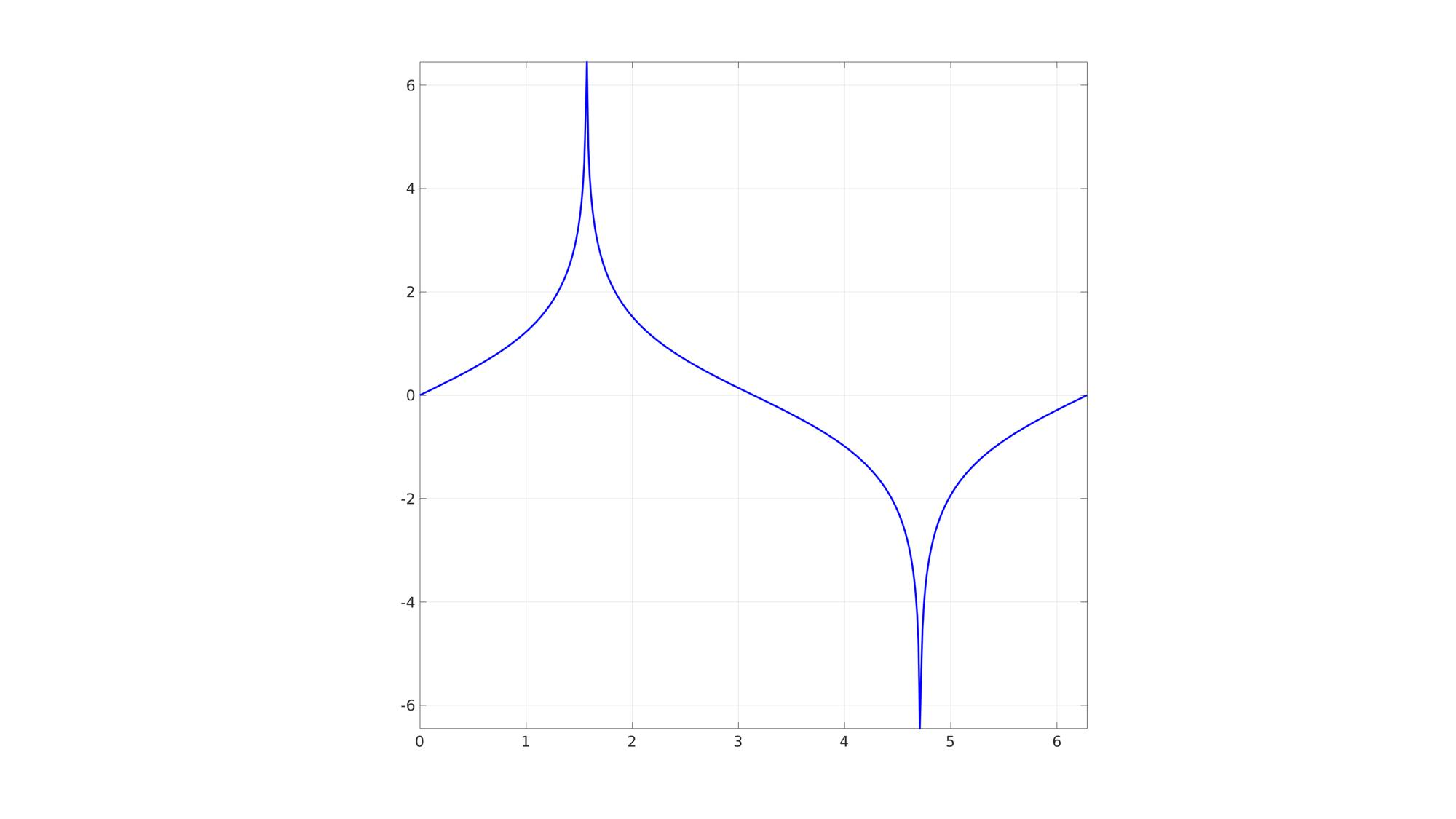# agd

Below is a demonstration of the features of the agd function

## Contents

```clear; close all; clc;
```

y=agd(x);

## Description

The output of is function y is the inverse Gudermannian of the input x. The inverse Gudermannian is defined as y=atanh(sin(x)). See also gd.

## Examples

Plot setttings

```lineWidth=3;
fontSize=25;
```

## Example 1: Computing the inverse Gudermannian

```x=linspace(0,2*pi,500); %A range of data
y=agd(x); %inverse Gudermannian
```

Visualize

```cFigure;
plot(x,y,'b-','LineWidth',lineWidth);
axis square; axis tight; grid on; box on;
set(gca,'FontSize',fontSize);
drawnow;
```GIBBON www.gibboncode.org

Kevin Mattheus Moerman, [email protected]